Input devices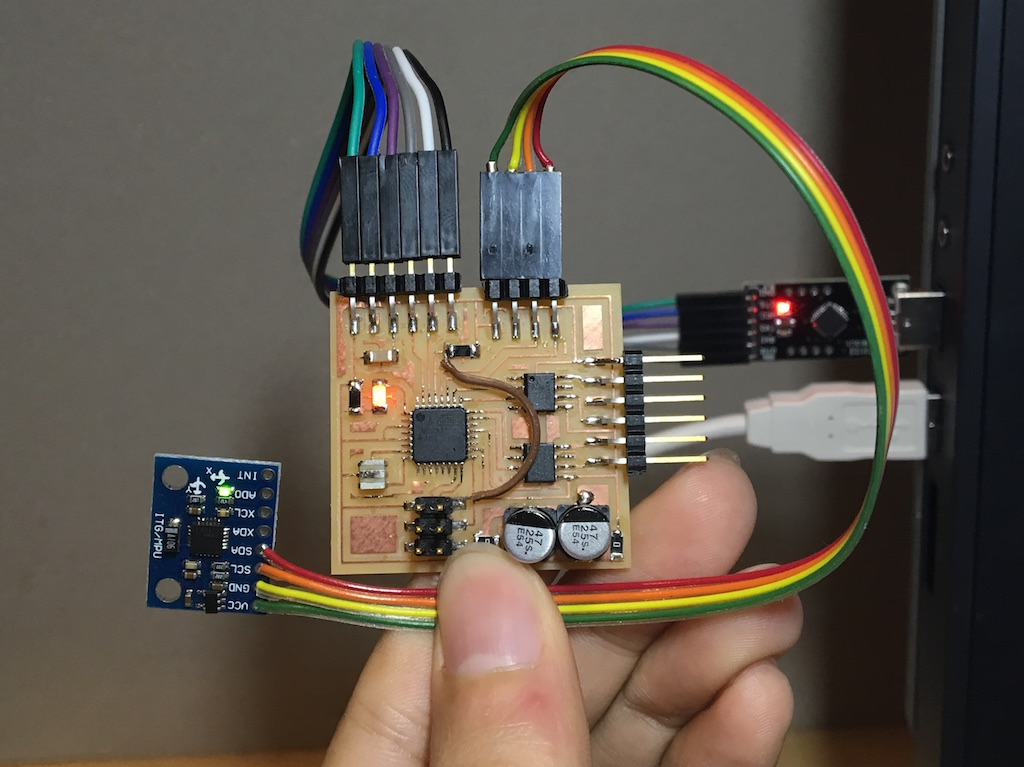This week I designed the circuit based on Atmega328P microcontroller to read IMU (inertial measurement unit). And I also designed the board to be able to drive brushless motors for next project. The microcontroller talks with the IMU unit through I2C interface. The 6 axis IMU breakout board was bought on amazon about \$3 which can read both the acceleration and rotation speed. The goal is to have the microcontroller read the IMU with I2C and pass the number to the computer through serial.The large capacitor is for filter high voltage fluctuations when it's used to control motors. And LED is added for easy debugging. There are two H bridges which I will use to drive motor and wireless power transfer.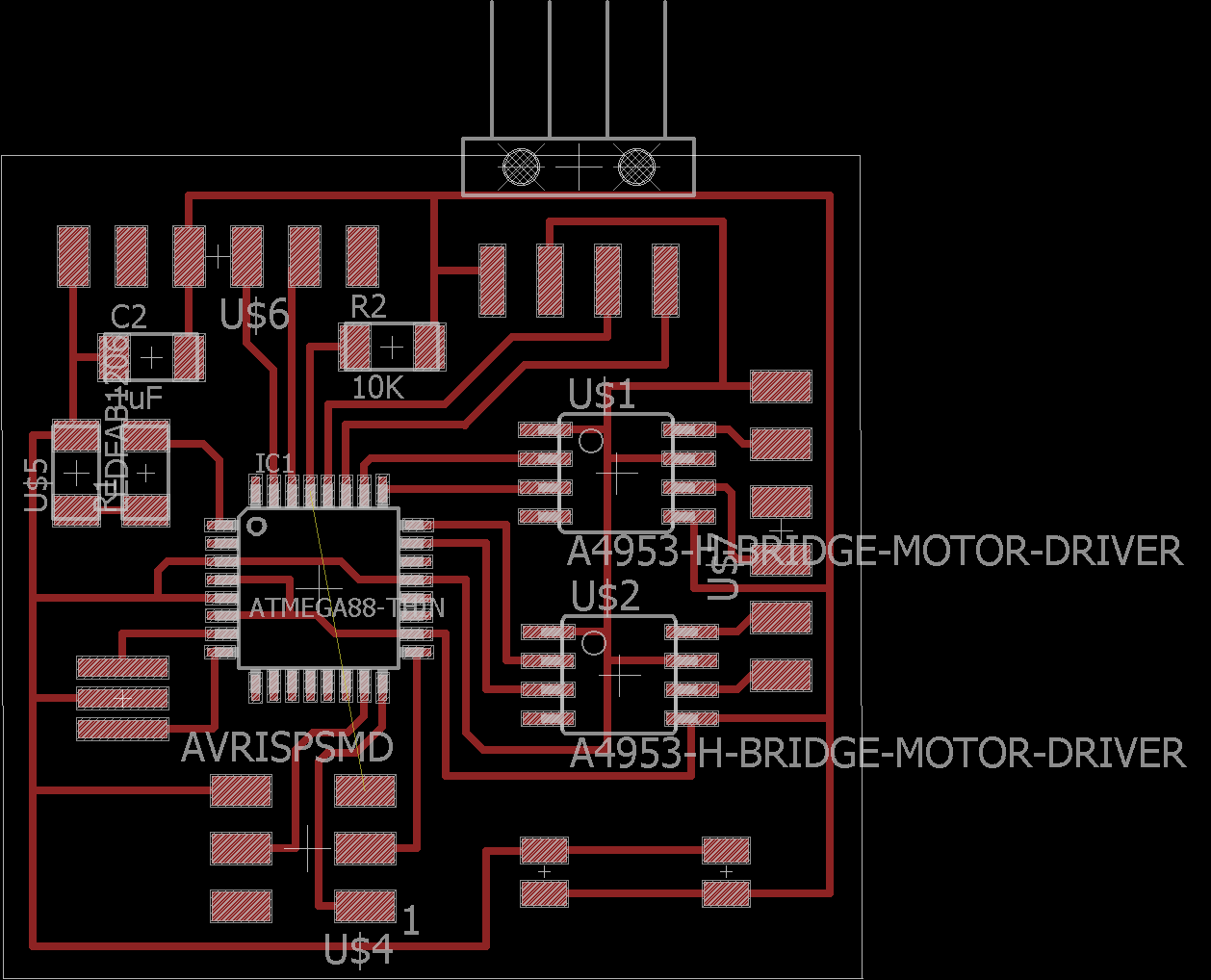On the IMU, the SCL and SDA are the clock and data line. The microcontroller serves as the master and IMU is the slave.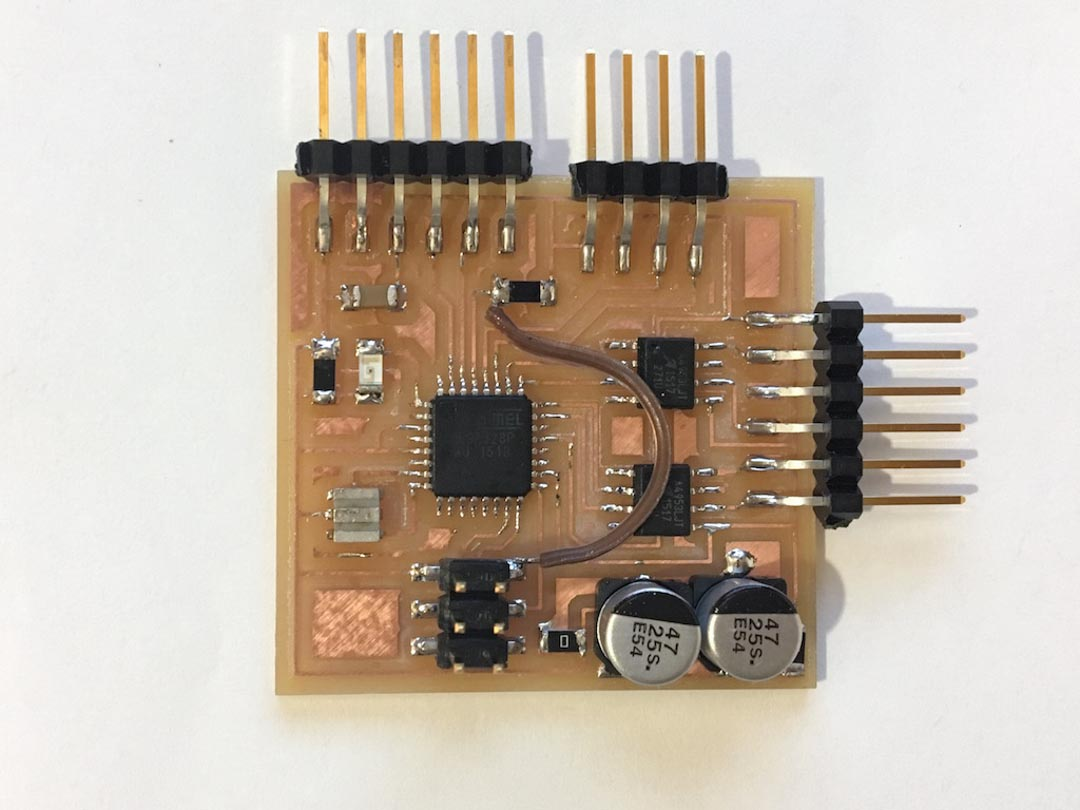Atmega328P already have I2C function build in the hardwire, which it calls it TWI (two wire interface). The following is the make file for it. Note the frequency is 20Mhz and the fuse is 0xD6 which sets external clock up to 20Mhz

``````
PROJECT=I2C
SOURCES=\$(PROJECT).c
MMCU=atmega328p
F_CPU = 20000000

CFLAGS=-mmcu=\$(MMCU) -Wall -Os -DF_CPU=\$(F_CPU)

\$(PROJECT).hex: \$(PROJECT).out
avr-objcopy -O ihex \$(PROJECT).out \$(PROJECT).c.hex;\
avr-size --mcu=\$(MMCU) --format=avr \$(PROJECT).out

\$(PROJECT).out: \$(SOURCES)
avr-gcc \$(CFLAGS) -I./ -o \$(PROJECT).out \$(SOURCES)

program-usbtiny: \$(PROJECT).hex
avrdude -p atmega328p -P usb -c usbtiny -U flash:w:\$(PROJECT).c.hex

program-usbtiny-fuses: \$(PROJECT).hex
avrdude -p atmega328p -P usb -c usbtiny -U lfuse:w:0xD6:m
``````

Below is the C code I wrote. The first part (separated by slashes) is for serial communication with the computer; the second part is to set up functions for I2C communication and the third part is to initialize, set options and read numbers from IMU. I used pointers to pass the number out from the read function. And as each reading is 16digit long, I used uint16 and read twice, first the high 8 digits and then the lower ones.

``````
#define F_CPU 20000000
#define BAUD 9600
#define BRC ((F_CPU/16/BAUD)-1)
#define MPU6050 0x68

#include <avr/io.h>
#include <stdio.h> // for serial
#include <util/delay.h>
#include <util/setbaud.h>
#include <util/twi.h>
#include <inttypes.h> // for printf uint16_t

void uart_init(void){
UBRR0L = BRC;
UBRR0H = (BRC >> 8); //Baud rate setting
UCSR0B |= (1 << RXEN0) | (1 << TXEN0); //RxTx enable
UCSR0C = (1 << UCSZ01) | (1 << UCSZ00); //8bit char
}

void uart_setchar(char c) {
loop_until_bit_is_set(UCSR0A, UDRE0); /* Wait until data register empty. */
UDR0 = c;
}

char uart_getchar(void) {
loop_until_bit_is_set(UCSR0A, RXC0); /* Wait until data exists. */
return UDR0;
}

int usart_putchar_printf(char var, FILE *stream) {
//if (var == '\n') usart_putchar('\r');
uart_setchar(var);
return 0;
}

static FILE mystdout = FDEV_SETUP_STREAM(usart_putchar_printf, NULL, _FDEV_SETUP_WRITE);
//define stream

///////////////////////////////////////////////////////////////////////////////////////////

void I2C_init(void){
//TWSR = 0x00;
TWBR = 0x5C;// TWI clock 100kHz, SCL frequency = F_CPU/(16 + 2(TWBR)*(TWSR prescale))
TWCR = (1 << TWEN);
}

void I2C_start(void){
TWCR = (1 << TWEN) | (1 << TWINT) |(1 << TWSTA);
//twi enable/clear int flag/start (master)
while ((TWCR & (1 << TWINT)) == 0);//? wait for TWINT to be 1?
//if ((TWSR & 0xF8) != TW_START) return -1;
//comfire start condition has been transmitted
}

void I2C_stop(void){
TWCR = (1 << TWEN) | (1 << TWINT) |(1 << TWSTO);
}

void I2C_write(uint8_t data){
TWDR = data;
TWCR = (1 << TWINT) | (1 << TWEN);
while ((TWCR & (1 << TWINT)) == 0);//? wait for TWINT to be 1
//if ((TWSR & 0xF8) != TW_MT_SLA_ACK) return -1;
//comfire start condition has been transmitted
}

TWCR = (1 << TWINT)|(1 << TWEN)|(1 << TWEA);//with acknowledge
while ((TWCR & (1 << TWINT)) == 0);
return TWDR;
}

TWCR = (1 << TWINT)|(1 << TWEN);//without acknowledge
while ((TWCR & (1 << TWINT)) == 0);
return TWDR;
}

uint8_t I2C_status(void)
{
uint8_t status;
status = TWSR & 0xF8;
return status;
}

///////////////////////////////////////////////////////////////////////////////////////////

uint8_t MPU_init(){
I2C_init();
I2C_start();
if (I2C_status() != TW_START) return -1;
I2C_write(((MPU6050 << 1) | TW_WRITE)); //TW_WRITE = 0 defined in twi.h
if (I2C_status() != TW_MT_SLA_ACK) return -1;
if (I2C_status() != TW_MT_DATA_ACK) return -1;
I2C_write(0);//set PWR_MGMT_1 to 0
if (I2C_status() != TW_MT_DATA_ACK) return -1;
I2C_stop();
_delay_ms(1);//allow time for stop to be sent
return 0;
}

I2C_init();
I2C_start();
if (I2C_status() != TW_START) return -1;
I2C_write(((MPU6050 << 1) | TW_WRITE)); //TW_WRITE = 0 defined in twi.h
if (I2C_status() != TW_MT_SLA_ACK) return -1;
if (I2C_status() != TW_MT_DATA_ACK) return -1;
I2C_write(u8data);//set value
if (I2C_status() != TW_MT_DATA_ACK) return -1;
I2C_stop();
_delay_us(10);//allow time for stop to be sent
return 0;
}

I2C_start();
if (I2C_status() != TW_START) return -1;
I2C_write(((MPU6050 << 1) | TW_WRITE)); //TW_WRITE = 0 defined in twi.h
if (I2C_status() != TW_MT_SLA_ACK) return -1;
if (I2C_status() != TW_MT_DATA_ACK) return -1;
I2C_stop();
_delay_us(10);//wait for data

I2C_start();
if (I2C_status() != TW_START) return -1;
if (I2C_status() != TW_MR_SLA_ACK) return -1;

*u16data = I2C_readACK() << 8; //significant 8 bits with acknowledge
*u16data |= I2C_readNACK(); //no ACK after the last bytes.
I2C_stop();
_delay_us(10);//wait for data
return 0;
}

int main(void) {
DDRD = (1 << PD3);
CLKPR = (1 << CLKPCE);
CLKPR = (0 << CLKPS3) | (0 << CLKPS2) | (0 << CLKPS1) | (0 << CLKPS0);

stdout = &mystdout;// setup stdio stream
uart_init();
//char chr;

//uint16_t data = 0;
uint16_t AcX;
uint16_t AcY;
uint16_t AcZ;
uint16_t GyroX;
uint16_t GyroY;
uint16_t GyroZ;
MPU_init();
MPU_write(0x1A, 3);
while (1) {
//PORTD ^= (1 << PD3);
//chr = uart_getchar();
//uart_setchar(chr);
printf("%" PRIu16 "\n",GyroX-32768);
PORTD ^= (1 << PD3);
_delay_ms(10);
}
return 0;
}
``````

The code runs successfully and I used the arduino serial plot to visualize the data. One tricky thing about serial here is the Tx on the microcontroller should go the Rx on the FTDI cable, and vise versa.Accelerometer test, AcY. The left one is changing the direction of the board to change the g force. The right one is shaking it.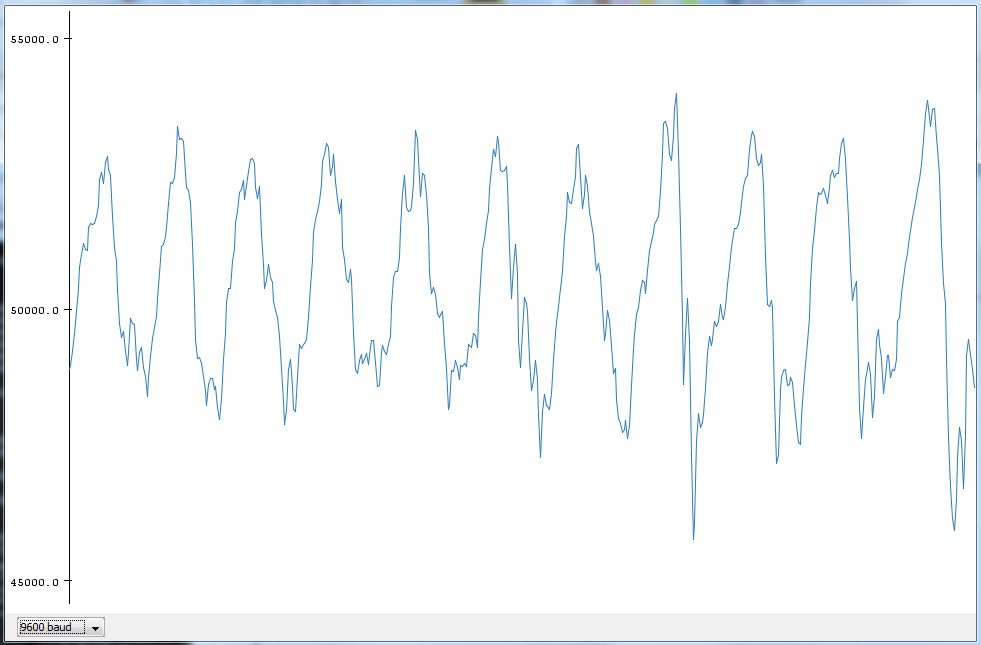Gyroscope test, GyroY,the left one is rotating it forth and back, the right one is with a touch and let it stablize.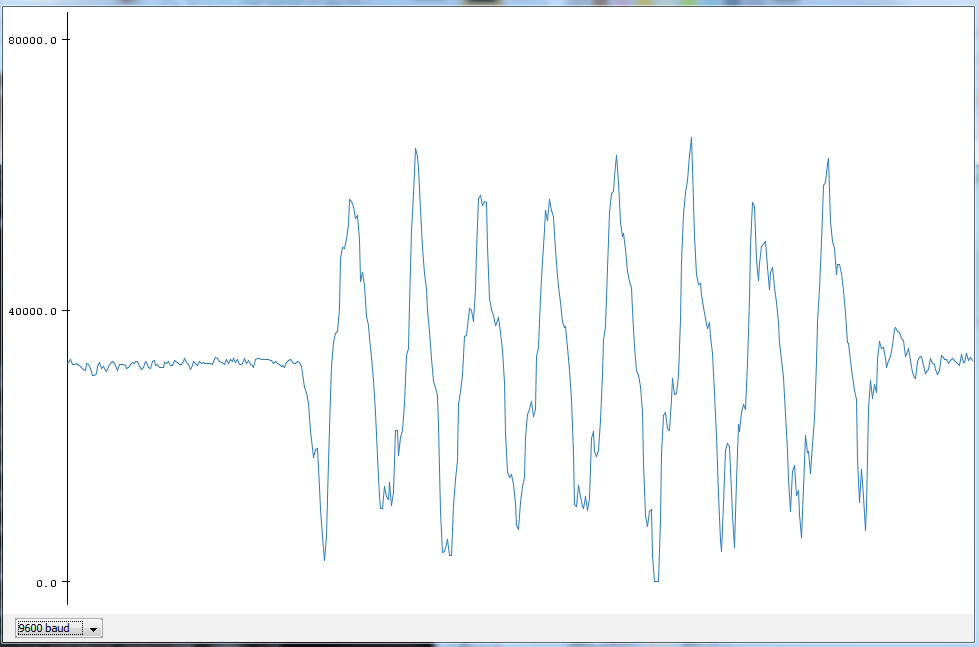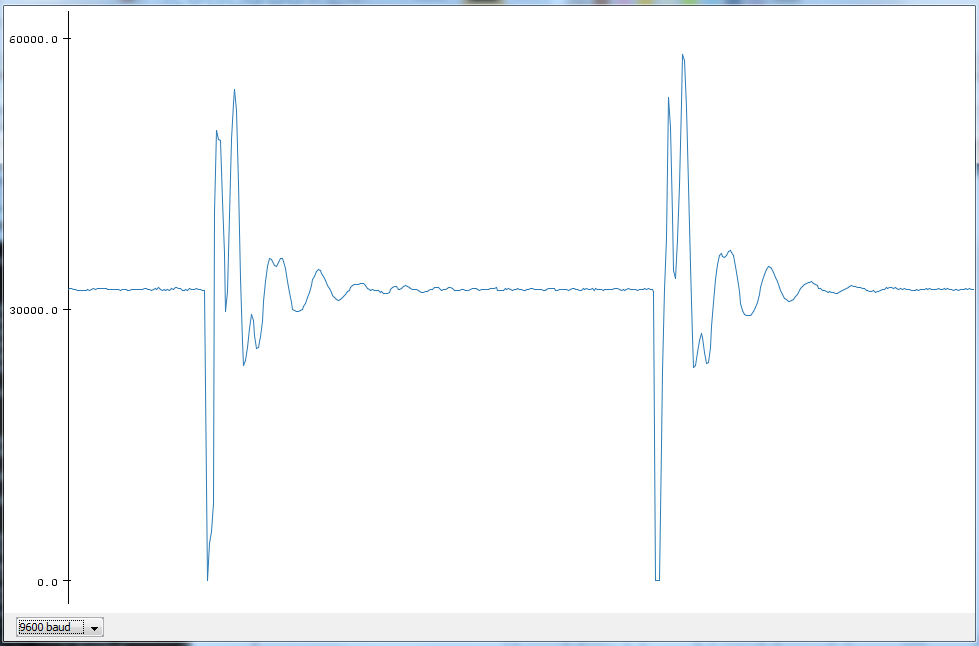``````
Xiaomeng.name=Xiaomeng

Xiaomeng.build.board=AVR_PRO
Xiaomeng.build.core=arduino

Xiaomeng.menu.cpu.8MHzatmega328=ATmega328 (5V, Internal 8 MHz, Optiboot 76k8)